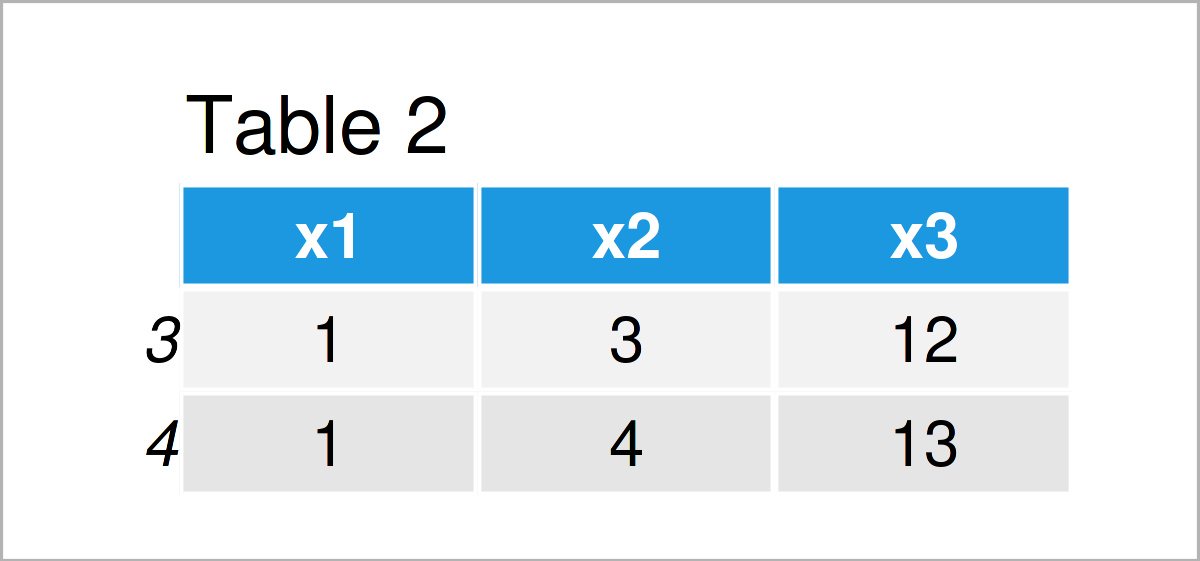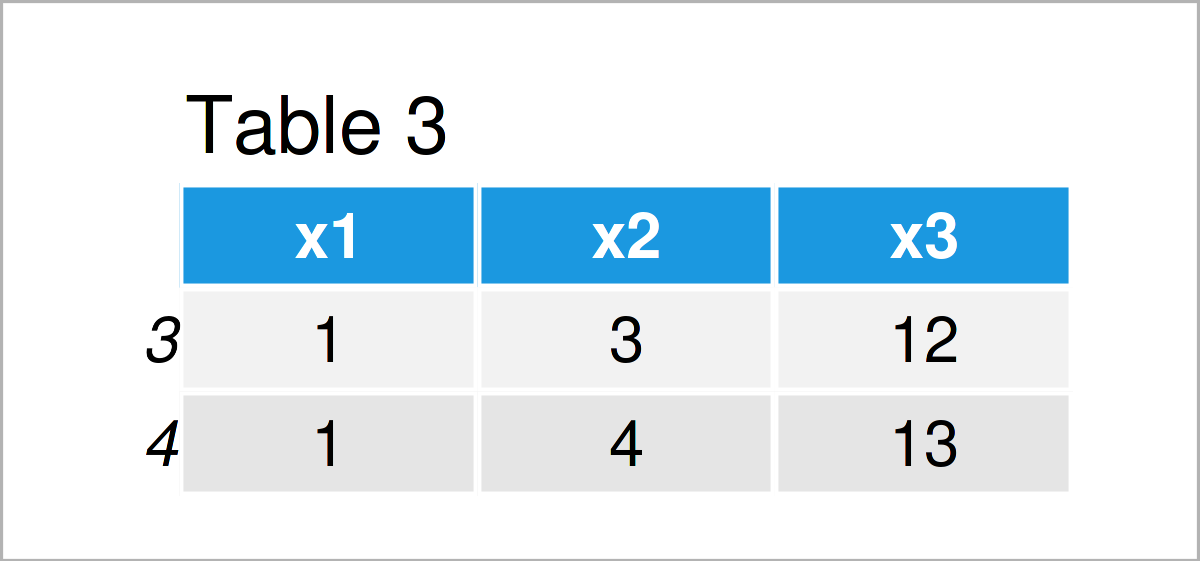# Remove Rows with NaN Values in R (3 Examples)

In this R programming tutorial you’ll learn how to drop data frame rows containing NaN values.

Let’s just jump right in…

## Introduction of Example Data

Let’s first create some example data in R:

```data <- data.frame(x1 = c(1, NaN, 1, 1, NaN),           # Create example data
x2 = c(1:4, NaN),
x3 = c(NaN, 11:14))
data                                                    # Print example data```As you can see based on Table 1, our example data is a data frame having five observations and three numerical columns. Some of the cells in our data are Not a Number (i.e. NaN).

## Example 1: Delete Rows Containing NaN Using na.omit() Function

The following R programming syntax demonstrates how to extract and remove NaN values from a data frame using the na.omit function.

Have a look at the following R code and its output:

```data_1 <- na.omit(data)                                 # Apply na.omit function
data_1                                                  # Print data without NaN rows```As shown in Table 2, we have managed to construct a new data frame object containing only rows without NaN values.

## Example 2: Delete Rows Containing NaN Using complete.cases() Function

In this example, I’ll illustrate how to use the complete.cases function to retain only rows with no NaN values:

```data_2 <- data[complete.cases(data), ]                  # Apply complete.cases function
data_2                                                  # Print data without NaN rows```As shown in Table 3, the previous R programming code has constructed exactly the same data frame as the na.omit function in Example 1.

Whether you prefer to use the na.omit function or the complete.cases function to remove NaN values is a matter of taste.

## Example 3: Delete Rows Containing NaN Using rowSums(), apply() & is.nan() Functions

In Example 3, I’ll explain how to use a combination of the rowSums, apply, and is.nan functions to keep only data frame rows without NaNs.

Have a look at the following syntax:

```data_3 <- data[rowSums(apply(data, 2, is.nan)) == 0, ]  # rowSums, apply & is.nan
data_3                                                  # Print data without NaN rows```As shown in Table 4, the previous code has managed to construct another version of our output data frame that looks exactly the same (i.e. no NaN values).

## Video, Further Resources & Summary

I have recently released a video on my YouTube channel, which explains the topics of this article. You can find the video below.

Besides the video, you might want to read the related articles on this website. I have published numerous posts about topics such as graphics in R, vectors, and extracting data.

You have learned in this tutorial how to remove and select data frame rows containing NaN values in the R programming language. Don’t hesitate to let me know in the comments, in case you have any further questions.

Subscribe to the Statistics Globe Newsletter